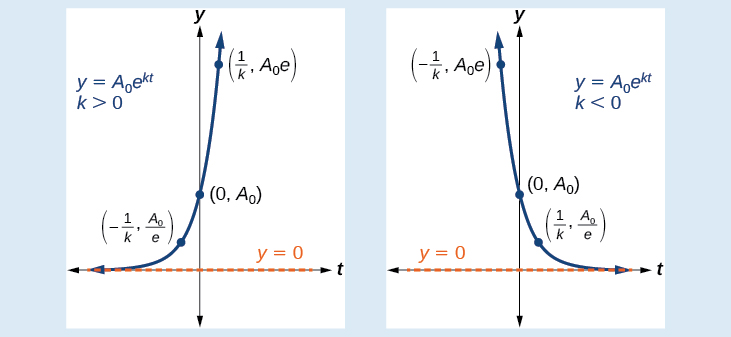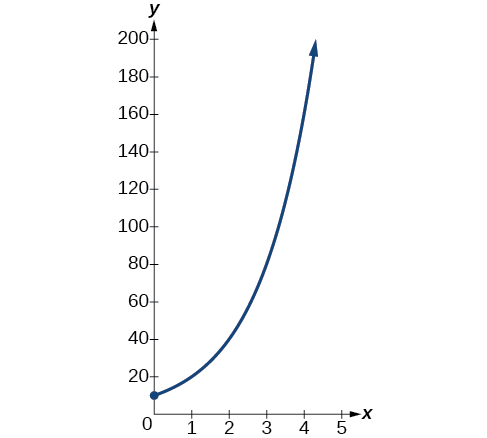# 6.7 Exponential and logarithmic models  (Page 2/16)

 Page 2 / 16

## Characteristics of the exponential function, y = A0e kt

An exponential function with the form $y={A}_{0}{e}^{kt}$ has the following characteristics:

• one-to-one function
• horizontal asymptote: $y=0$
• domain:
• range: $\left(0,\infty \right)$
• x intercept: none
• y-intercept: $\left(0,{A}_{0}\right)$
• increasing if $k>0$ (see [link] )
• decreasing if $k<0$ (see [link] )An exponential function models exponential growth when k > 0 and exponential decay when k < 0.

## Graphing exponential growth

A population of bacteria doubles every hour. If the culture started with 10 bacteria, graph the population as a function of time.

When an amount grows at a fixed percent per unit time, the growth is exponential. To find ${A}_{0}$ we use the fact that ${A}_{0}$ is the amount at time zero, so ${A}_{0}=10.$ To find $k,$ use the fact that after one hour $\left(t=1\right)$ the population doubles from $10$ to $20.$ The formula is derived as follows

so $k=\mathrm{ln}\left(2\right).$ Thus the equation we want to graph is $\text{\hspace{0.17em}}y=10{e}^{\left(\mathrm{ln}2\right)t}=10{\left({e}^{\mathrm{ln}2}\right)}^{t}=10·{2}^{t}.\text{\hspace{0.17em}}$ The graph is shown in [link] .The graph of y = 10 e ( ln 2 ) t

## Half-life

We now turn to exponential decay . One of the common terms associated with exponential decay, as stated above, is half-life , the length of time it takes an exponentially decaying quantity to decrease to half its original amount. Every radioactive isotope has a half-life, and the process describing the exponential decay of an isotope is called radioactive decay.

To find the half-life of a function describing exponential decay, solve the following equation:

$\frac{1}{2}{A}_{0}={A}_{o}{e}^{kt}$

We find that the half-life depends only on the constant $k$ and not on the starting quantity ${A}_{0}.$

The formula is derived as follows

Since $t,$ the time, is positive, $k$ must, as expected, be negative. This gives us the half-life formula

$t=-\frac{\mathrm{ln}\left(2\right)}{k}$

Given the half-life, find the decay rate.

1. Write $A={A}_{o}{e}^{kt}.$
2. Replace $A$ by $\frac{1}{2}{A}_{0}$ and replace $t$ by the given half-life.
3. Solve to find $k.$ Express $k$ as an exact value (do not round).

Note: It is also possible to find the decay rate using $k=-\frac{\mathrm{ln}\left(2\right)}{t}.$

## Finding the function that describes radioactive decay

The half-life of carbon-14 is 5,730 years. Express the amount of carbon-14 remaining as a function of time, $t.$

This formula is derived as follows.

The function that describes this continuous decay is $f\left(t\right)={A}_{0}{e}^{\left(\frac{\mathrm{ln}\left(0.5\right)}{5730}\right)t}.$ We observe that the coefficient of $t,$ $\frac{\mathrm{ln}\left(0.5\right)}{5730}\approx -1.2097×{10}^{-4}$ is negative, as expected in the case of exponential decay.

The half-life of plutonium-244 is 80,000,000 years. Find function gives the amount of carbon-14 remaining as a function of time, measured in years.

$f\left(t\right)={A}_{0}{e}^{-0.0000000087t}$

The formula for radioactive decay is important in radiocarbon dating , which is used to calculate the approximate date a plant or animal died. Radiocarbon dating was discovered in 1949 by Willard Libby, who won a Nobel Prize for his discovery. It compares the difference between the ratio of two isotopes of carbon in an organic artifact or fossil to the ratio of those two isotopes in the air. It is believed to be accurate to within about 1% error for plants or animals that died within the last 60,000 years.

By the definition, is such that 0!=1.why?
(1+cosA+IsinA)(1+cosB+isinB)/(cos@+isin@)(cos$+isin$)
hatdog
Mark
how we can draw three triangles of distinctly different shapes. All the angles will be cutt off each triangle and placed side by side with vertices touching
bsc F. y algebra and trigonometry pepper 2
given that x= 3/5 find sin 3x
4
DB
remove any signs and collect terms of -2(8a-3b-c)
-16a+6b+2c
Will
Joeval
(x2-2x+8)-4(x2-3x+5)
sorry
Miranda
x²-2x+9-4x²+12x-20 -3x²+10x+11
Miranda
x²-2x+9-4x²+12x-20 -3x²+10x+11
Miranda
(X2-2X+8)-4(X2-3X+5)=0 ?
master
The anwser is imaginary number if you want to know The anwser of the expression you must arrange The expression and use quadratic formula To find the answer
master
The anwser is imaginary number if you want to know The anwser of the expression you must arrange The expression and use quadratic formula To find the answer
master
Y
master
master
Soo sorry (5±Root11* i)/3
master
Mukhtar
2x²-6x+1=0
Ife
explain and give four example of hyperbolic function
What is the correct rational algebraic expression of the given "a fraction whose denominator is 10 more than the numerator y?
y/y+10
Mr
Find nth derivative of eax sin (bx + c).
Find area common to the parabola y2 = 4ax and x2 = 4ay.
Anurag
y2=4ax= y=4ax/2. y=2ax
akash
A rectangular garden is 25ft wide. if its area is 1125ft, what is the length of the garden
to find the length I divide the area by the wide wich means 1125ft/25ft=45
Miranda
thanks
Jhovie
What do you call a relation where each element in the domain is related to only one value in the range by some rules?
A banana.
Yaona
a function
Daniel
a function
emmanuel
given 4cot thither +3=0and 0°<thither <180° use a sketch to determine the value of the following a)cos thither
what are you up to?
nothing up todat yet
Miranda
hi
jai
hello
jai
Miranda Drice
jai
aap konsi country se ho
jai
which language is that
Miranda
I am living in india
jai
good
Miranda
what is the formula for calculating algebraic
I think the formula for calculating algebraic is the statement of the equality of two expression stimulate by a set of addition, multiplication, soustraction, division, raising to a power and extraction of Root. U believe by having those in the equation you will be in measure to calculate it
Miranda

#### Get Jobilize Job Search Mobile App in your pocket Now!ByByBy Angelica LitoBy Edgar DelgadoBy Brooke DelaneyBy Brooke DelaneyBy Cath YuBy OpenStaxBy Joli JuliannaBy OpenStaxBy Yacoub JayoghliBy Brooke Delaney